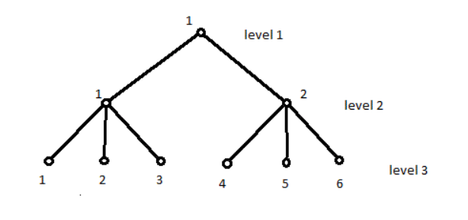# Not Too HardtechboyReplay of Intra AUST Prog...
Limits 1s, 512 MB

Showmik is very popular nowadays. Junior coders always seek help from him. He also wants them to improve their skills. So for them he has given a problem which is not too easy and again not too hard. He is generating a tree which has the following properties:

1. Each node takes 1 second to be created.
2. Each node will have $m$ child nodes. $m$ will be equal to (current level + 1).
3. Each node will be marked with a number starting from left. Number starts with 1.He will give a random number $N$. They have to find the level number and mark number of the node being created at $N$-th second.

## Input

First line of input contains a single number $T$ ($T \le 10000$). $T$ test cases follows. Each case has a single number $N$ ($1 \le N \le 10^{16}$) which indicates the $N$-th second.

## Output

For each test case, print answer as the format “Case x: y z” without quotation. Here, $x$ indicates the case number and $y$, $z$ indicate the desired results.

## Sample

InputOutput
4
5
21
63
1326509874132650
Case 1: 3 2
Case 2: 4 12
Case 3: 5 30
Case 4: 18 948498053512337

Tree is basically a graph which is connected and there is no cycle. There is only one root node and each node may have zero or more child nodes.ConstructiveuDebug

### Submit